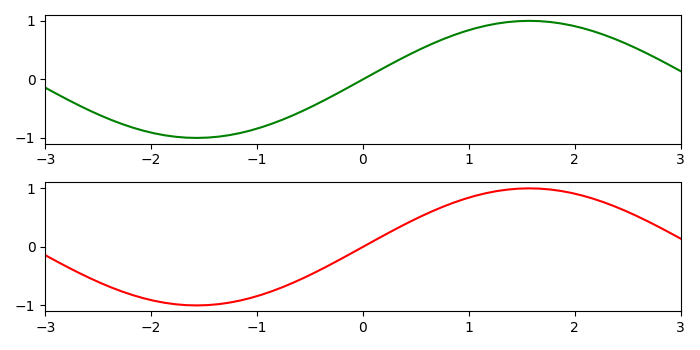# What is the difference betweent set_xlim and set_xbound in Matplotlib?

set_xlim − Set the X-axis view limits.

set_xbound − Set the lower and upper numerical bounds of the X-axis.

To set the xlim and xbound, we can take the following steps −

• Using subplots(2), we can create a figure and a set of subplots. Here, we are creating 2 subplots.

• Create x and y data points using numpy.

• Use axis 1 to plot x and y data points using plot() method.

• Set x limit using set_xlim() method.

• Use axis 2 to plot x and y data points using plot() method.

• Sex xbound using set_xbound() method.

• To display the figure, use show() method.

## Example

import numpy as np
from matplotlib import pyplot as plt
plt.rcParams["figure.figsize"] = [7.00, 3.50]
plt.rcParams["figure.autolayout"] = True
fig, axes = plt.subplots(2)

x = np.linspace(-5, 5, 100)
y = np.sin(x)

axes.plot(x, y, c='g')
axes.set_xlim(-3, 3)

axes.plot(x, y, c='r')
axes.set_xbound(-3, 3)

plt.show()

## OutputUpdated on: 11-May-2021

248 Views